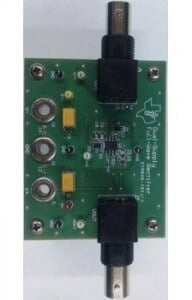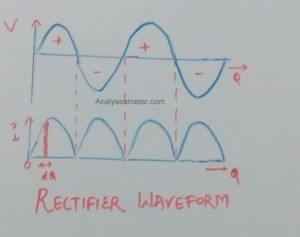# Full wave Rectifier Efficiency Equation and Applications

Full wave rectifier basically uses both half cycles of the applied AC voltage and converts an AC voltage into a pulsating DC voltage. In full wave rectification, one diode conducts during one half-cycle while other conducts during the other half cycle of the applied AC voltage. It has some advantages over half wave rectifier like it has high average output DC voltage and has much fewer ripples resulting in the smoother output waveform. In our previous article, we explain Rectifier, half wave rectifier efficiency, diodes, p-n junction etc in detail. In this article, we are going to explain the mathematical expression of an efficiency of Full wave rectifier equation and its applications etc.## Full-wave Rectifier Equations

The efficiency of a full wave rectifier is defined as the ratio of DC power output to the applied input AC power. Therefore, it is given as;

η = DC Power Output / AC power input

### (i) Mathematical derivation of Full wave rectifier efficiency:

Let the v = Vm sin θ be the AC voltage to be rectified, rf be the forward resistance of crystal diode and RL be the load resistance. The diagram given below shows the input voltage wave and rectified output wave.The instantaneous value of current is given by the equation:

i = v / (rf + RL)

As we know,

v = Vm sin θ

Therefore,

i = Vm sin θ / (rf + RL)

When sin θ = 1, then current = maximum. Therefore,

Im = Vm / (rf + RL)

Where,

i = Im sin θ

Since output is obtained across RL , therefore

D.C power output = Idc2 RL

= *Iav2 RL

Where,

Iav = ʃ (i dθ) / π   ….. (i)

Integrate equation (i) from 0 to π,

Iav = (1 / π) * ʃ Im sin θ dθ

= (Im / π) * ʃ sin θ dθ

= (Im / π) [ – cos θ ]

= (Im / π) [ -(-1-1)]

= 2 (Im / π)

= (2Im / π)

Therefore, DC power output is given as,

Pdc = Idc2 RL = (2Im / π)2 RL

And AC power input is given as,

Pac =Irms2 (rf + RL)

Where,

** Irms =  ʃ (i2 dθ) / π ….. (ii)

= √ (1 / π) * ʃ Im2 sin2 θ dθ

= √ (Im2 / π) * ʃ ( 1- cos 2θ)/ 2  dθ

= √ (Im2 / 2π) * [ ʃ dθ – ʃ cos 2θ dθ ]

= √ (Im2 / 2π) * [[θ] – [sin 2θ / 2]]

= √ (Im2 / 2π) * [π – 0]

= Im / √ 2

Therefore, AC power input is given as,

Pac =Irms2 (rf + RL)

=( Im / √2)2 (rf + RL)

As we know,

Rectifier Efficiency (η) = Pdc / Pac

Put the values of Pdc and Pac from above equations, therefore,

η =[ (2Im / π)2 * RL ] / [( Im / √2)2 * (rf + RL)]

= 0.812 RL / (rf + RL)

= 0.812 / (1+ rf /RL)

If rf is neglected as compared to RL then the efficiency of the rectifier is maximum. Therefore,

η max =0.812 = 81.2%

### Form factor:

It is defined as the ratio of RMS value of the output voltage to the average value of the output voltage. Its equation can be given as:

Form Factor = RMS value of the output voltage / average value of the output voltage

= (Vm / √2) / (2 Vm / π) = π / 2 √2

Form Factor = 1.11

### Peak Factor:

It is defined as the ratio of the peak value of the output voltage to the RMS value of the output voltage. Its equation can be given as:

Peak factor = Peak value of the output voltage / RMS value of the output voltage

= Vm / (Vm / √2)

Peak Factor = √2

## Applications of Full wave Rectifier:

Full wave rectifier is of two types; centre tap and bridge rectifier. Both these rectifiers are used for following purposes depends upon the requirement. Following of full wave rectifier applications are:

• It can be used to detect the amplitude of modulated radio signal.
• It can be used to supply polarised voltage in welding.
• It is mainly used in power supply circuits.Hi readers, Ajay Kumar is graduated in Electronics and Communication Engineering is responsible for running this website. With the knowledge, he has gained, he tries to provide the readers as much of it as he can.

### 13 thoughts on “Full wave Rectifier Efficiency Equation and Applications”

1.Its too gud explanation

•Ajay Kumar

Thanks Varun for commenting. We are happy that we helped you.

2.Thanks sir

•Gupta Himanshi

3.thank u so much

•Ajay Kumar

Welcome Suman,
We are glad that you find our website helpful. Share the article with your friends too. This keeps us encourage to produce quality and helpful content. 🙂

4.Rishitha

It’s really a very useful n helpful site……..satisfied with it………

•Hi Rishitha,
Thanks for stopping by to comment. We are glad that you find our website useful. If you have any question you can ask in a comment.

5.Sylvester kobby

Thanks very much for the lesson

6.Raj kumar

and keep providing such best explanations.

•Thank you Raj, for investing your time and commenting. These comments keep us motivated.

7.choudhary nikhil

satisfying…..with this topic
keep growing….

•